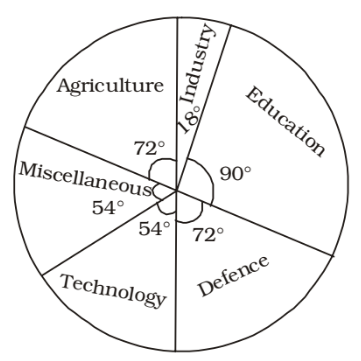## Introduction to Data Interpretation

#### Data Interpretation

Direction: The following pie-chart shows the marks scored by a student in different subjects - viz. Physics (Ph), Chemistry (Ch), Mathematics (M), Social Science (SS) and English (E) in an examination. Assuming that total marks obtained for the examination is 810. Answer the questions given below.1. The marks obtained in Mathematics and Chemistry exceed the marks obtained in Physics and Social Science by

1. Sum of corresponding angles of Maths and Chemistry
= 90 + 70 = 160°
Sum of corresponding angles of Physics and Social Science
= 85 + 55 = 140°
Difference = 20°
∵ 360° ≡ 810

 ∴ 20° ≡ 810 × 20 = 45 360

##### Correct Option: D

Sum of corresponding angles of Maths and Chemistry
= 90 + 70 = 160°
Sum of corresponding angles of Physics and Social Science
= 85 + 55 = 140°
Difference = 20°
∵ 360° ≡ 810

 ∴ 20° ≡ 810 × 20 = 45 360

1. The difference of marks between Physics and Chemistry is same as that between

1. Difference of corresponding angles :
Physics and Chemistry = 85 – 70 = 15°
Chemistry and social science = 70 – 55 = 15°

##### Correct Option: A

Difference of corresponding angles :
Physics and Chemistry = 85 – 70 = 15°
Chemistry and social science = 70 – 55 = 15°

Direction: Following figure is Pie-chart representing item-wise cost of manufacturing certain product.
Study the chart and answer the questions.1. The difference of cost of material and direct expenses is

1. Difference of respective angles = 144° – 43.2° = 100.8°
∴ Required difference

 = 96000 × 100.8° = \$ 26880 360°

##### Correct Option: D

Difference of respective angles = 144° – 43.2° = 100.8°
∴ Required difference

 = 96000 × 100.8° = \$ 26880 360°

1. Total manufacturing cost is \$ 96,000. Then, cost of labour is

1. ∵ 360° ≡ \$ 96000

 ∴ 115.2° ≡ 96000 × 115.2 360°

= \$ 30720

##### Correct Option: A

∵ 360° ≡ \$ 96000

 ∴ 115.2° ≡ 96000 × 115.2 360°

= \$ 30720

Direction: The Pie Chart shows the expenditure of a country on various sports during a particular year.
Study the graph and answer the questions.1. Study the graph & answer the questionIn a certain country, allocations to various sectors of the yearly budget per \$ 1000 crores are represented by this pie-diagram. The expenditure (in \$) on Agriculture is

1. Expenditure on agriculture sector

 = 72° × 1000 360

= \$ 200 crores

##### Correct Option: D

Expenditure on agriculture sector

 = 72° × 1000 360

= \$ 200 crores